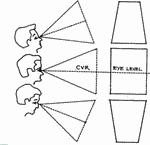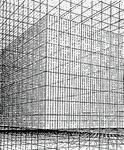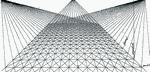# Perspective Projection

## perspective projection

[pər′spek·tiv prə′jek·shən]
(graphic arts)
A projection of points by straight lines drawn through them from some given point to an intersection with the plane of projection.

## Perspective projection

A method of projection in which a three-dimensional object can be represented by projecting points upon a picture plane using straight lines converging at a fixed point, representing the eye of the viewer.### center of vision

A point representing the intersection of the central axis of vision and the picture plane in linear perspective drawing.

### central visual axis

The sightline, which is perpendicular to the picture plane, indicating the direction in which the viewer is looking.

### cone of vision

The field of vision radiating outwardly from the eye of the viewer in a more or less conical shape along the central visual axis.### ground line

A horizontal line representing the intersection of the ground plane and the picture plane.

### ground plane

A horizontal plane of reference in linear perspective from which vertical measurements can be taken; usually it is the plane supporting the object depicted or the one on which the viewer stands.

### horizon line

A horizontal line in linear perspective representing the intersection of the picture plane and a horizontal plane through the eye of the viewer.

### measuring line

Any line coincident with or parallel to the picture plane, as the ground line, on which accurate measurements can be taken.

### one-point perspective

A rendition of an object with a principal face parallel to the picture plane; all horizontal lines parallel to the picture plane remain as is, and all other horizontal lines converge to a preselected vanishing point.### picture plane

An imaginary transparent plane, coexistent with the drawing surface, on which the image of a three-dimensional object is projected and on which all lines can be measured and drawn to exact scale.### station point

A fixed point in space representing a single eye of the viewer.

### three-point perspective

A perspective of an object with all faces oblique to the picture plane; the three sets of parallel lines converge to three different vanishing points: one left, one right, and one above or below the horizon line.### two-point perspective

A perspective of an object having two faces oblique to the picture plane. The vertical lines parallel to the picture plane remain vertical, and two horizontal sets of parallel lines oblique to the picture plane appear to converge to two vanishing points: one to the left and one to the right.### vanishing point

A point toward which receding parallel lines appear to converge, located at the point where a sight line parallel to the set of lines intersects picture plane.## Perspective Projection

a particular case of azimu-thal cartographic projections. A perspective projection can be obtained by projecting a sphere onto a picture plane tangent to a pole of the sphere; the projection is carried out by rays from an eye point lying on a line perpendicular to the plane and passing through the center of the sphere. Examples of perspective projections are the gnomonic projection, the stereographic projection, and external projections, where the eye point is outside the sphere at a finite distance, or at an infinite distance—in the latter case it is called orthographic projection.

## perspective projection

The projection of points by straight lines drawn through them from some given point to an intersection with the plane of projection.
References in periodicals archive ?
Next, the 3D model/image is reconstructed from a pair of two-dimensional (2D) images to visualize the perspective projection in a 3D space.
Radviz can be explained as a combination of a perspective projection and a linear mapping with the viewpoint at the origin and the view plane being a simplex.
A fair approximation for most conventional narrow-angle and even wide-angle lenses is the pinhole camera model which fulfilling the perspective projection described by following formula: r = f tan[THETA], where [THETA] refers to the angle between the optical axis and the incoming ray, r is the distance between the imagine point and the principal point and f is a focal length.
From the characteristics of the perspective projection transformation, points in a straight line in the scene are still in a straight line after perspective projection transformation.
The perspective projection of eye and camera can be approximately looked as a pinhole model.
Another parameter of perspective projection to receive attention from investigators is the display geometric field of view (GFOV) and its relation to field of view (FOV).
The object-screen transformation is usually either an orthographic projection (sometimes called a parallel plane projection) or a (central) perspective projection.
The viewer-centered perspective, as well as the projections used for stereo, are derived from the off-axis perspective projection .

Site: Follow: Share:
Open / Close